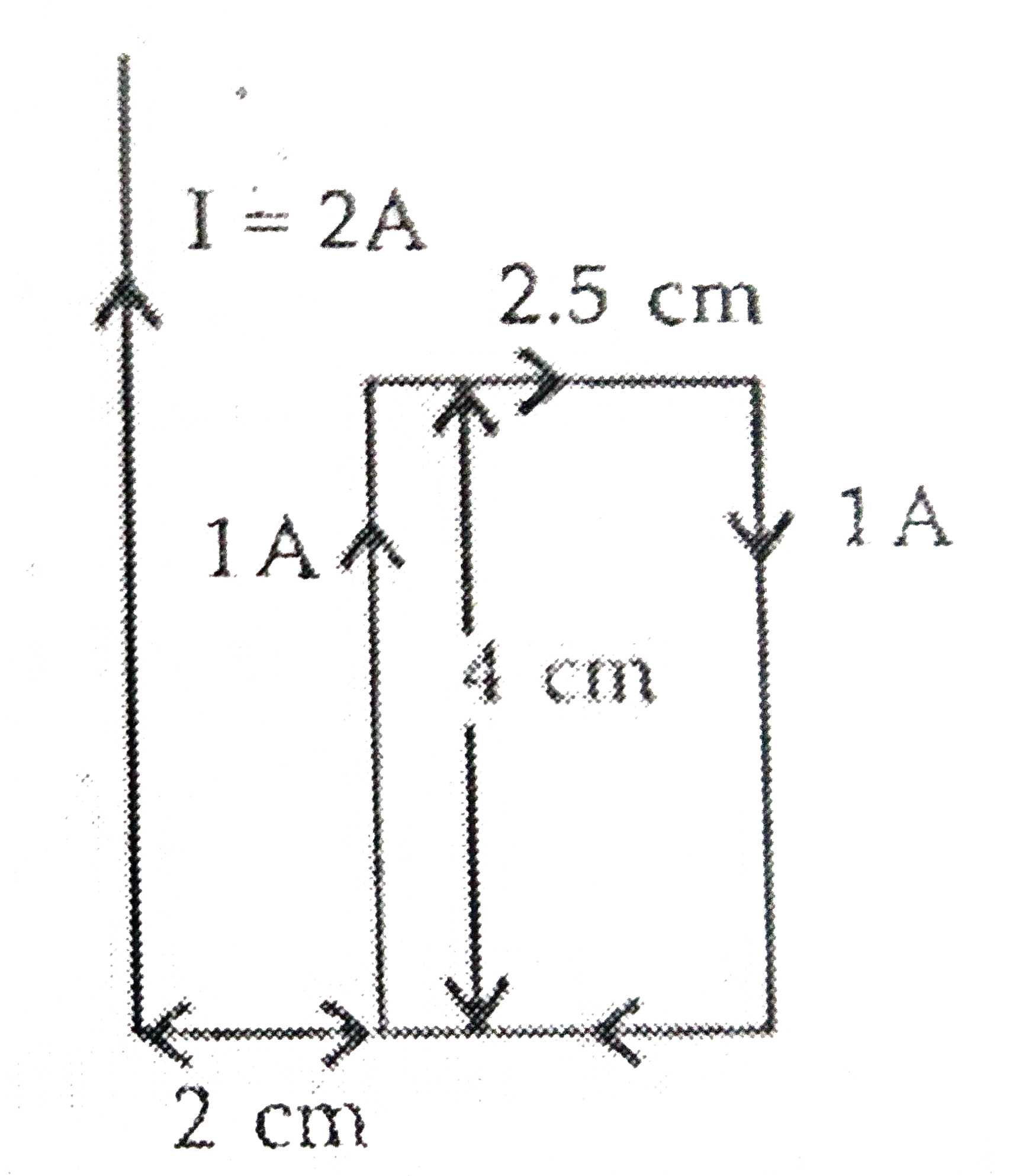Question

# A rectangular loop of wire of size 2.5cm×4cm carries a steady current of 1A. A straight long wire carrying 2 A current is kept near the loop as shown. If the loop and the wire are coplanar, find (i) the torque acting on the loop and (ii) the magnitude and direction of the force on the loop due to the current carrying wire.Khareedo DN Pro and dekho sari videos bina kisi ad ki rukaavat ke!

Step by step solution by experts to help you in doubt clearance & scoring excellent marks in exams.

Transcript

question is a rectangular loop of wire of side 2.5 cm into 4 cm carries a Steady Current of 1 ampere so here we can see current flowing in this wire is clockwise on a long wire carrying current is kept near the loop shown it is kept at a distance of 2 CM if the loop and the wire ko cleaners in the same plane find the torque on the loop so we can see magnetic moment of this group is let's across into the plane of the people as we know the magnetic moment is equal to N into I into the area is calculated that is inside and we can say your current flowing in the to a long + Y direction due to this current we can say
magnetic field along this cross-sectional area will be into the plane of the paper that is my Right Hand Thumb Rule inside hence we can say that the current carrying loop kis given a cross b vector and since M and b vector a parallel to each other so Emraan Hashmi becomes men2b into sin 0 degree which is equal to zero is equal to zero so for the first part we can set on is zero coming to the second part the magnitude and direction of the force on the loop due to the current carrying the why so can we see that letter se latest name this loop as P Q R to continue carrying current in same direction experience a force so we know that force act
per unit length is given as you're not into one in 2025 into so if we let set take this current as to a we can see the force experienced by the us so we can see that this PS1 experiences and same direction current carrying wire experiences a force which is given by the magnitude is equal to as we know that force between two wires let se can be written as motor unite 2 into 2 by 3 into this becomes as Mu not value if we write value of Mu not as 4.2 10 power minus 7 divided by 25 this becomes 2 into 10 power minus 7 we can see current in first wire is to a
the second wire is 1 a / distance between the virus is of 2 cm and length of the wire we can see PS is given as B if i m to cm 4 cm and to cancel don't need to convert into ammeter is this comes to be 8 into 10 power minus 7 Newton this is the force on the wi a vs in a similar manner we can see the wire lead to a let's say they are flowing in opposite direction current hence in this case force on this via QR will be signature which will be let selatan own QR is given as in a similar manner of Mu not is equal to
4.2 10 power minus 7 25 becomes 2 into 10 power minus 7 into 12 a 2-1 / B Ed distance between you are wine and the to a point is 2 cm and 2.5 CM X 4.5 cm and the length of the is equal to 4 cm and this can be written this becomes 9.2 so 16 into two which is equal to 32 19 into 10 power we can ship -7 Newton as we can see the force on the wire PS is greater than that on the we can say let us consider an element the length of PQ so the magnetic field due to this tour
length PQ which is along p2q direction plus the magnetic field on this let se will be inside soap this magnetic field cross magnetic field due to a let se exerts a force elemental force on this elemental stripped long + y axis just opposite to that PQ let's say we elements same length as opposed to it SR we can say the elemental length comes Along r2s along the direction of current magnetic field due to a will be cross due to this week and it's a magnetic force will be a long minus y axis bf now we can see this DF and this DF of same magnitude and just opposite
direction of the cancel out each other so the net force will be due to PS and Q R and S P S is of Greater magnitude so the net force can be written as let se along minus x sixes even by the magnitude FPS - if you are so here we can see that the net will be equal to along minus x minus of f u r and along -66 status - is this comes out as 8 - 32 / 9 into 10 power minus 7 Newton of -1 am now 97232 is 49 into 10 power minus 7 of minus ICAP on simplification this comes as
936 so I can see here 4.44 into 10 power minus 7 Newton minus of ICAP so this is the net force experienced by the ullu

Related Videos